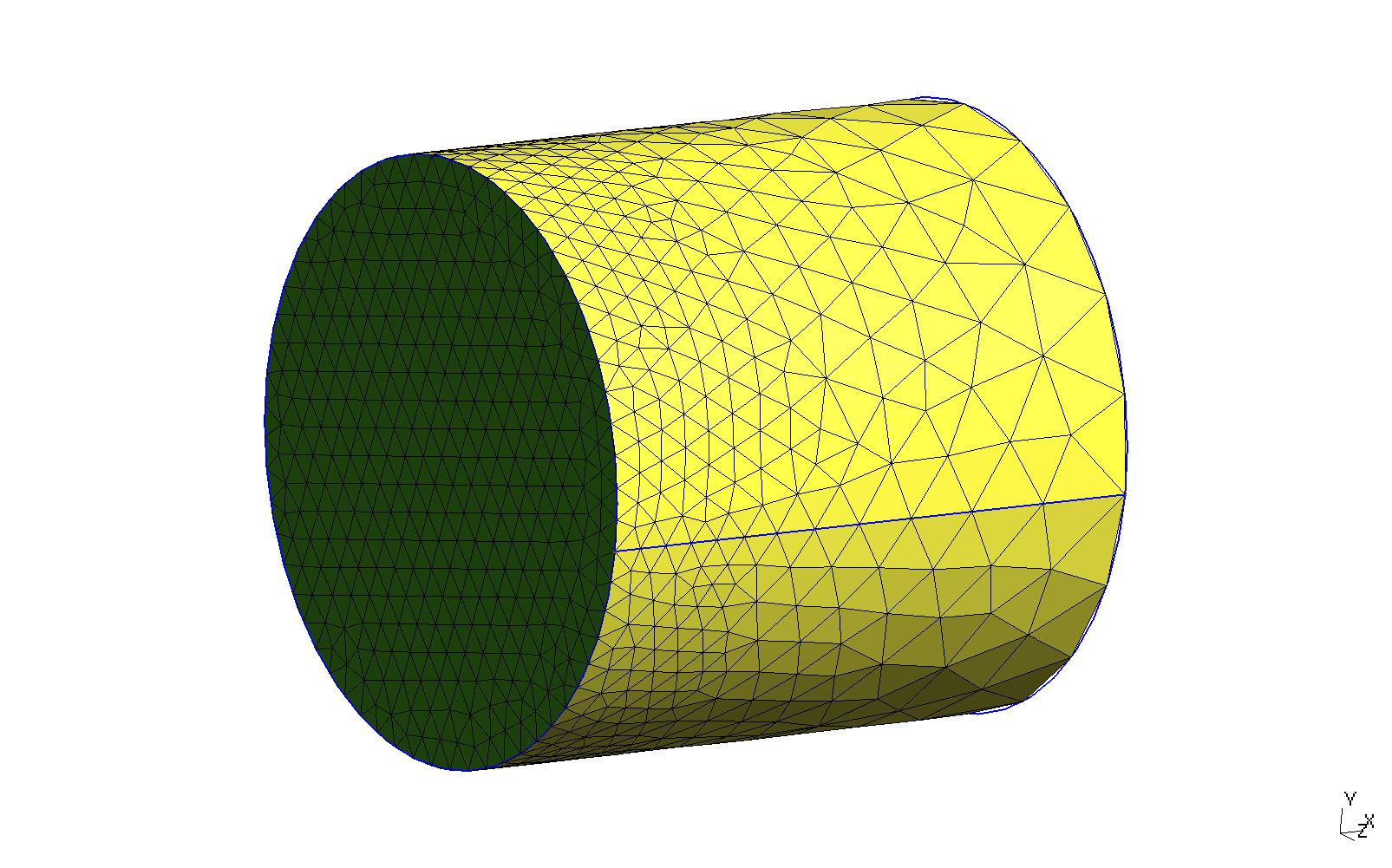# Heat conduction

2023-12-02

Abstract. Check out the thermal tutorial.

# 1 Thermal slabs

## 1.1 One-dimensional linear

Solve heat conduction on the slab x \in [0:1] with boundary conditions

\begin{cases} T(0) = 0 & \text{(left)} \\ T(1) = 1 & \text{(right)} \\ \end{cases}

and uniform conductivity. Compute T\left(\frac{1}{2}\right).

• The input written in a self-evident English-like dialect
• Syntactic sugared plain-text ASCII file
• Simple problems (like this one) need simple inputs
• FeenoX follows the UNIX rule of simplicity
• Output is 100% user-defined
• No PRINT no output
• Feenox follows the UNIX rule of silence
• There is no node at x=1/2=0.5!
• FeenoX knows how to interpolate
• Mesh separated from problem
• The geometry comes from a Git-friendly .geo
Point(1) = {0, 0, 0};          // geometry:
Point(2) = {1, 0, 0};          // two points
Line(1) = {1, 2};              // and a line connecting them!

Physical Point("left") = {1};  // groups for BCs and materials
Physical Point("right") = {2};
Physical Line("bulk") = {1};   // needed due to how Gmsh works

Mesh.MeshSizeMax = 1/3;        // mesh size, three line elements
Mesh.MeshSizeMin = Mesh.MeshSizeMax;
• UNIX rule of composition
• The actual input file is a Git-friendly .fee
PROBLEM thermal 1D    # tell FeenoX what we want to solve
k = 1                 # set uniform conductivity
BC left  T=0          # set fixed temperatures as BCs
BC right T=1          # "left" and "right" are defined in the mesh
SOLVE_PROBLEM         # we are ready to solve the problem
PRINT T(1/2)          # ask for the temperature at x=1/2
$gmsh -1 slab.geo [...] Info : 4 nodes 5 elements Info : Writing 'slab.msh'... [...]$ feenox thermal-1d-dirichlet-uniform-k.fee
0.5
$ # 2 Non-dimensional transient heat conduction on a cylinder Let us solve a dimensionless transient problem over a cylinder. Conductivity and heat capacity are unity. Initial condition is a linear temperature profile along the x axis: T(x,y,z,0) = x The base of the cylinder has a prescribed time and space-dependent temperature T(0,y,z,t) = \sin( 2\pi \cdot t) \cdot \sin( 2\pi \cdot y) The other faces have a convection conditions with (non-dimensional) heat transfer coefficient h=0.1 and T_\text{ref} = 1.Locally-refined cylinder for a transient thermal problem. PROBLEM thermal 3D READ_MESH cylinder.msh end_time = 2 # final time [ non-dimensional units ] # the time step is automatically computed # initial condition (if not given, stead-state is computed) T_0(x,y,z) = x # dimensionless uniform and constant material properties k = 1 kappa = 1 # BCs BC hot T=sin(2*pi*t)*sin(2*pi*y) BC cool h=0.1 Tref=1 SOLVE_PROBLEM # print the temperature at the center of the base vs time PRINT %e t T(0,0,0) T(0.5,0,0) T(1,0,0) WRITE_MESH temp-cylinder.msh T IF done PRINT "\# open temp-anim-cylinder.geo in Gmsh to create a quick rough video" PRINT "\# run temp-anim-cylinder.py to get a nicer and smoother video" ENDIF $ gmsh -3 cylinder.geo
[...]
Info    : Done optimizing mesh (Wall 0.624941s, CPU 0.624932s)
Info    : 1986 nodes 10705 elements
Info    : Writing 'cylinder.msh'...
Info    : Done writing 'cylinder.msh'
Info    : Stopped on Fri Dec 24 10:35:32 2021 (From start: Wall 0.800542s, CPU 0.896698s)
$feenox temp-cylinder-tran.fee 0.000000e+00 0.000000e+00 5.000000e-01 1.000000e+00 1.451938e-04 4.406425e-07 5.000094e-01 9.960851e-01 3.016938e-04 9.155974e-07 5.000171e-01 9.921274e-01 5.566768e-04 1.689432e-06 5.000251e-01 9.862244e-01 8.565589e-04 2.599523e-06 5.000292e-01 9.800113e-01 1.245867e-03 3.780993e-06 5.000280e-01 9.728705e-01 1.780756e-03 5.404230e-06 5.000176e-01 9.643259e-01 2.492280e-03 7.563410e-06 4.999932e-01 9.545723e-01 3.428621e-03 1.040457e-05 4.999538e-01 9.436480e-01 [...] 1.978669e+00 -6.454358e-05 1.500891e-01 2.286112e-01 1.989334e+00 -3.234439e-05 1.500723e-01 2.285660e-01 2.000000e+00 1.001730e-14 1.500572e-01 2.285223e-01 # open temp-anim-cylinder.geo in Gmsh to create a quick rough video # run temp-anim-cylinder.py to get a nicer and smoother video$ python3 temp-anim-cylinder.py
Info    : 1986 nodes
Info    : 10612 elements
0 1 0.0
0.01 12 0.8208905327853042
0.02 15 0.8187351216040447
0.03 17 0.7902629708599855
[...]
Info    : Writing 'temp-cylinder-smooth-198.png'...
Info    : Done writing 'temp-cylinder-smooth-198.png'
199
Info    : Writing 'temp-cylinder-smooth-199.png'...
Info    : Done writing 'temp-cylinder-smooth-199.png'
all frames dumped, now run
ffmpeg -framerate 20 -f image2 -i temp-cylinder-smooth-%03d.png temp-cylinder-smooth.mp4
to get a video
$ffmpeg -y -f image2 -i temp-cylinder-smooth-%03d.png -framerate 20 -pix_fmt yuv420p -c:v libx264 -filter:v crop='floor(in_w/2)*2:floor(in_h/2)*2' temp-cylinder-smooth.mp4 [...]$


# 3 Non-dimensional transient heat conduction with time-dependent properties

Say we have two cubes of non-dimensional size 1\times 1 \times 1, one made with a material with unitary properties and the other one whose properties depend explicitly ony time. We glue the two cubes together, fix one side of the unitary material to a fixed zero temperature and set a ramp of temperature between zero and one at the opposite end of the material with time-varying properties.

This example illustrates how to

1. assign different material properties to different volumes
2. give time-dependent material properties and boundary conditions
3. plot temperatures as function of time at arbitrary locations on space
PROBLEM thermal 3D

end_time = 50
# initial condition (if not given, stead-state is computed)
# T_0(x,y,z) = 0

# dimensionless uniform and constant material properties
k_left = 0.1+0.9*heaviside(t-20,20)
rho_left = 2-heaviside(t-20,20)
cp_left = 2-heaviside(t-20,20)

# dimensionless uniform and constant material properties
k_right = 1
rho_right = 1
cp_right = 1

# BCs
BC zero  T=0
BC ramp  T=limit(t,0,1)
BC side  q=0

SOLVE_PROBLEM

PRINT t T(0,0,0) T(0.5,0,0) T(1,0,0) T(1.5,0,0) T(2,0,0)
$gmsh -3 two-cubes.geo [...]$ feenox two-cubes-thermal.fee > two-cubes-thermal.dat
\$